# CEOE Advanced Math: Integration Techniques Chapter Exam

Exam Instructions:

Choose your answers to the questions and click 'Next' to see the next set of questions. You can skip questions if you would like and come back to them later with the yellow "Go To First Skipped Question" button. When you have completed the practice exam, a green submit button will appear. Click it to see your results. Good luck!

### Page 1

#### Question 1 1. Evaluate the following integral.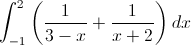#### Question 2 2. Integrate the following.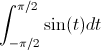#### Question 3 3. Evaluate the integral.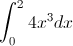#### Question 4 4. What is the volume made by revolving f(x) around the x-axis between x=0 and x=1?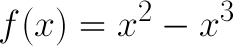#### Question 5 5. Evaluate the following integral.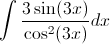### Page 2

#### Question 6 6. Evaluate the integral.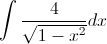#### Question 7 7. Evaluate the following integral.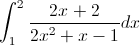#### Question 8 8. Evaluate the integral.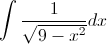#### Question 9 9. Evaluate the following integral.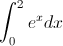#### Question 10 10. Simplify the following integral.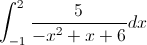### Page 3

#### Question 11 11. Factor the fraction below.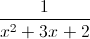#### Question 12 12. Evaluate the following.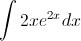#### Question 14 14. Factor the fraction below.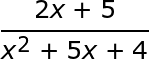#### Question 15 15. Integrate the following.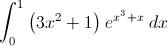### Page 4

#### Question 16 16. Evaluate and solve the following integral.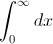#### Question 17 17. Find z, the shaded area in the graph below.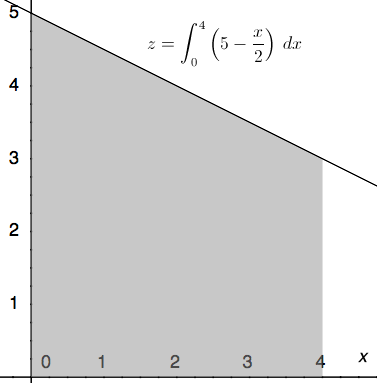#### Question 18 18. Evaluate the following.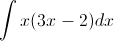#### Question 19 19. Evaluate the integral.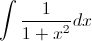#### Question 20 20. Evaluate the following integral.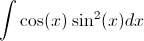### Page 5

#### Question 21 21. Evaluate the following integral.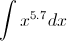#### Question 22 22. Evaluate the following integral.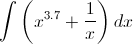#### Question 23 23. Evaluate and solve the following integral.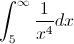#### Question 24 24. Evaluate the following integral.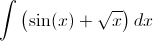#### Question 25 25. What is the volume made by revolving f(x) around the x-axis between x=0 and x=1?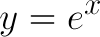### Page 6

#### Question 26 26. Integrate the following.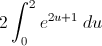#### Question 27 27. Integrate the following.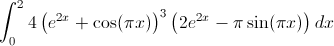#### Question 28 28. What is the volume made by revolving the area between g(x) and f(x) around the x-axis between x=0 and x=1?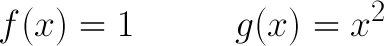#### Question 29 29. Factor the fraction below.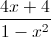#### Question 30 30. Evaluate the following integral.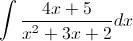#### CEOE Advanced Math: Integration Techniques Chapter Exam Instructions

Choose your answers to the questions and click 'Next' to see the next set of questions. You can skip questions if you would like and come back to them later with the yellow "Go To First Skipped Question" button. When you have completed the practice exam, a green submit button will appear. Click it to see your results. Good luck!

Support